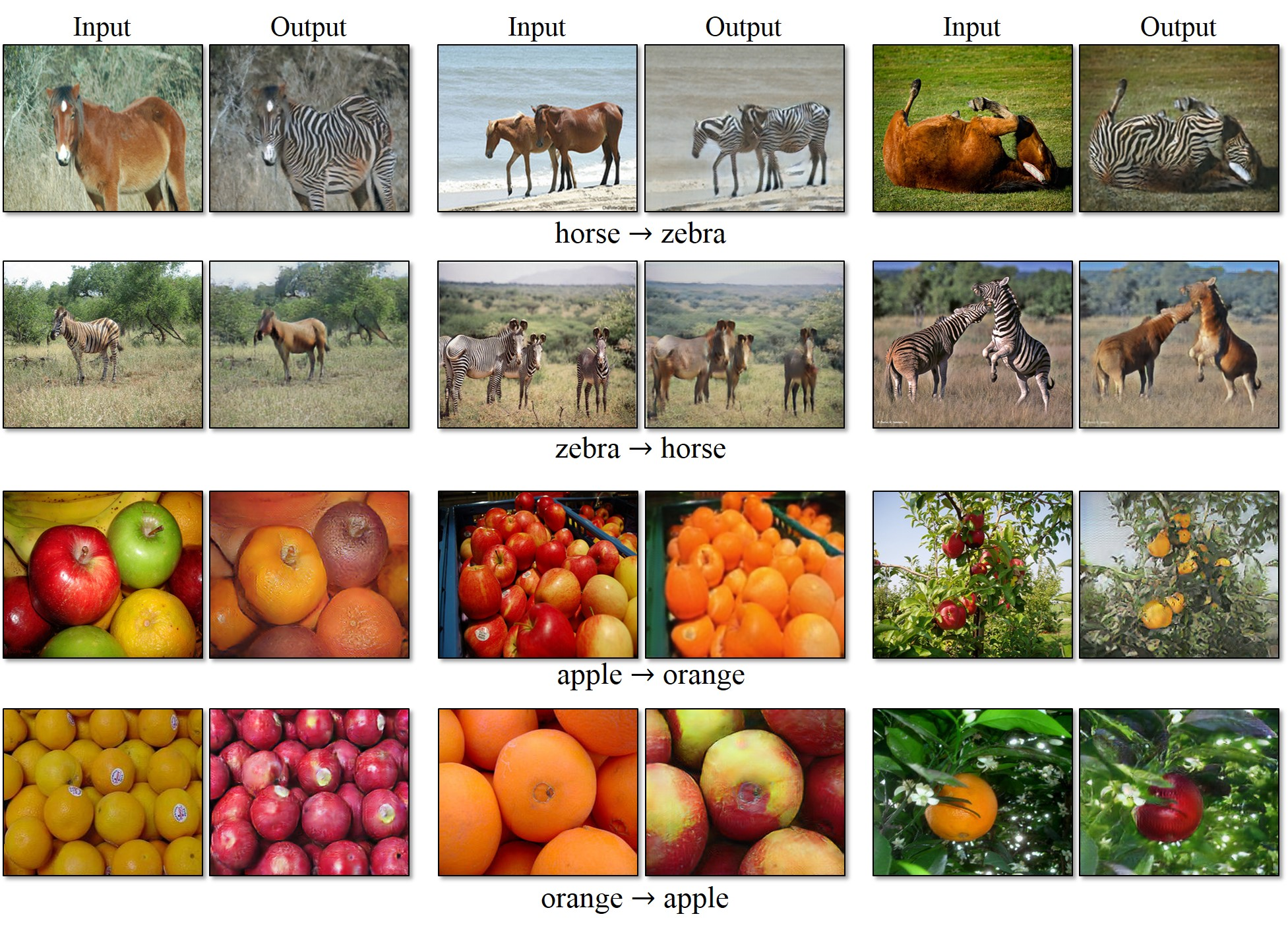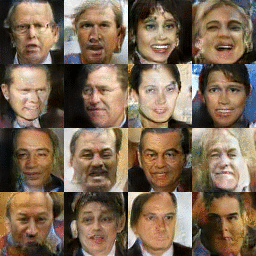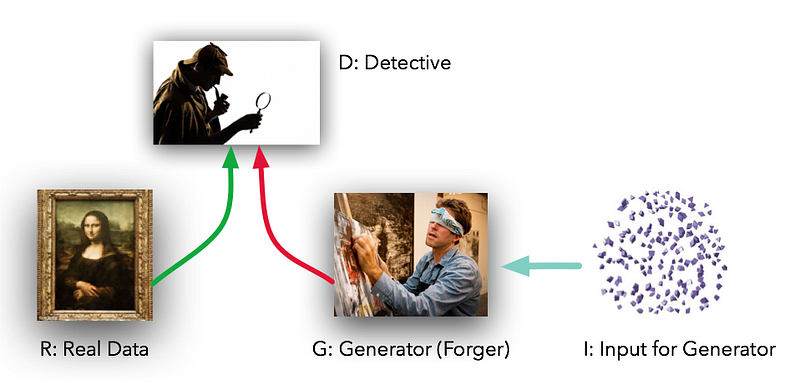# Problem statement of Image Translation### Learn $G: (S \rightarrow T)$

$G$ that convert an image of source domain $S$ to an image of target domain $T$

### Paired and Unpaired dataset### Image Translation: $S$ and $T$ are pair-wise labeled in dataset### Image Translation: $S$ and $T$ are not pair-wised in dataset# Before, Style Transfer (NeuralArt) was prominentBut it largely depends on textual information of an target style\begin{equation}
\min_G \max_D V(D,G) = \mathbf{E}_{x\sim p_{\text{data}}(x)} [\log D(x)] + \mathbf{E}_{z\sim p_{z}}(z)[\log (1 - D(G(z)))].
\end{equation}

# Two major problems of Image Translation

1. Convert to which domain?
• learn which "$G: (S \rightarrow T)$"?
2. How to learn the dataset?
• how to properly form dataset?
• pair-wise Supervised? or Unsupervised?

### Today, presenting SOTA of Image Translation papers of

• pix2pix: Image-to-Image Translation with Conditional Adversarial Networks (CVPR2017)
• Domain Transfer Network: Unsupervised Cross-Domain Image Generation (ICLR2017)
• CycleGAN & DiscoGAN: CycleGAN: Unpaired Image-to-Image Translation using Cycle-Consistent Adversarial Networks (ICCV2017) & DiscoGAN: Learning to Discover Cross-Domain Relations with Generative Adversarial Networks (ICML2017)
• BicycleGAN: Toward Multimodal Image-to-Image Translation (NIPS2017)